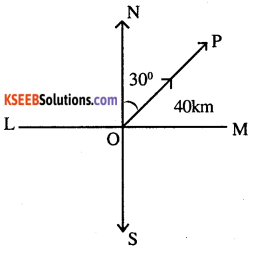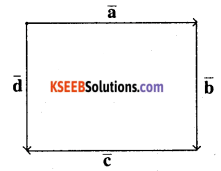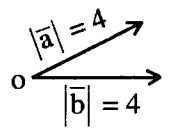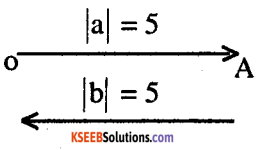# 2nd PUC Maths Question Bank Chapter 10 Vector Algebra Ex 10.1

Students can Download Maths Chapter 10 Vector Algebra Ex 10.1 Questions and Answers, Notes Pdf, 2nd PUC Maths Question Bank with Answers helps you to revise the complete Karnataka State Board Syllabus and score more marks in your examinations.

## Karnataka 2nd PUC Maths Question Bank Chapter 10 Vector Algebra Ex 10.1

### 2nd PUC Maths Vector Algebra NCERT Text Book Questions and Answers Ex 10.1

Question 1.
Represent graphically a displacement of 40 km, 30° east of north.
Graphically a displacement of 40 km 30° east of north.
OP = 40 km
∠NOP = 30°Question 2.
Classify the following measures as scalars and Vectors.
(i) 10 kg
(ii) 2 meters north-west
(iii) 40°
(iv) 40 watt
(v) 10-19 coulomb
(vi) 20 m/s2
(i) 10 kg – Scalar
(ii) 2 meters north-west – Vector
(iii) 40° – Scalar
(iv) 40 watt – Scalar
(v) 10-19 coulomb- Scalar
(vi) 20 m/s2 – VectorQuestion 3.
Classify the following as scalar and vector quantities.
(i) time period
(ii) distance
(iii) force
(iv) velocity
(v) work done
(i) time period  – Scalar
(ii) distance – Scalar
(iii) force – Vector
(iv) velocity – Vector
(v) work done – Scalar

Question 4.
In Fig 10.6 (a square), identify the following vectors.(i) Coinitial
(ii) Equal
(iii) Collinear but not equal
(i) Coinitial $$\overline{\mathbf{a}} \text { and } \overline{\mathbf{d}}$$ are co-initial vectors

(ii) Equal
$$\overline{\mathbf{b}} \text { and } \overline{\mathbf{d}}$$ are equal vectors

(iii) Collinear but not equal
$$\overline{\mathbf{a}} \text { and } \overline{\mathbf{c}}$$ are collinear but not equalsQuestion 5.
Answer the following as true or false.
(i) $$\overline{\mathbf{a}} \text { and }-\overline{\mathbf{a}}$$ are collinear.
(ii) Two collinear vectors are always equal in magnitude.
(iii) Two vectors having same magnitude are collinear.
(iv) Two collinear vectors having the same magnitude are equal.
(i) $$\overline{\mathbf{a}} \text { and }-\overline{\mathbf{a}}$$ are collinear – True